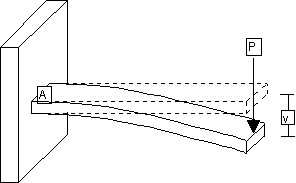# Given a point-load at the end of a wall-mounted cantilever beam, what is the shear stress at the wall?The shear stress at the wall should be positive P.
Let me know if my anser is not correct.

Hi @Weijun_Zhang, positive P would be the shear force in this case I believe. If we’re thinking about the shear force diagram that would be the case…but shear stress should be a force applied over an area.

In this case it would be:

Shear Stress= +P / v

where P = shear force, v = cross-sectional area.

Here’s a reference equation I found from Google:

Great! Thanks for pointing this out. So the shear stress should be P/A based on the reference equation.

@Weijun_Zhang That’s correct! Feel free to comment on any other posts you may be confused about, and we will try our best to answer.